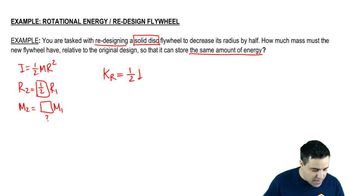Start typing, then use the up and down arrows to select an option from the list.
3:42 minutes
Problem 9c
Textbook Question

# A uniform sphere with mass 28.0 kg and radius 0.380 m is rotating at constant angular velocity about a stationary axis that lies along a diameter of the sphere. If the kinetic energy of the sphere is 236 J, what is the tangential velocity of a point on the rim of the sphere?Verified Solution
This video solution was recommended by our tutors as helpful for the problem above.
67views